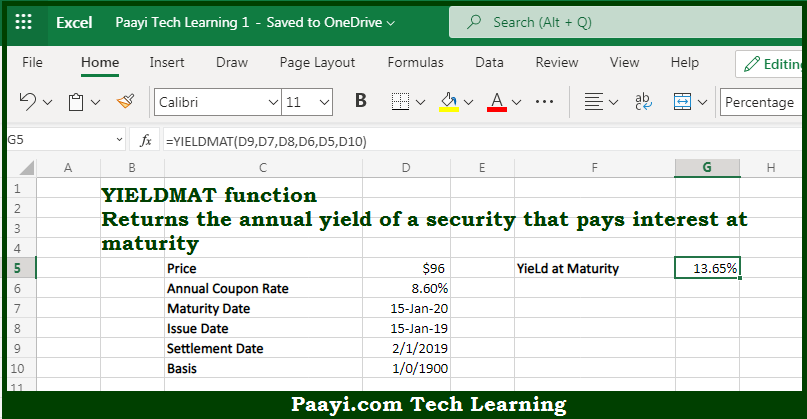# Learn How to Use Microsoft Excel YIELDMAT Function

Written by | 0 Comments | 632 Views

In this article, you will learn how to use the Microsoft Excel YIELDMAT function and its prime function in Microsoft Excel. You will also get to know the Microsoft Excel YIELDMAT function return value and syntax with the help of some examples.

Microsoft Excel YIELDMAT Function

The main purpose of the Microsoft Excel YIELDMAT function is to get the annual yield for the security interest at maturity. That implies, with the help of the YIELDMAT function you can able to return the annual yield of a security that pays interest at maturity. So, with the help of the YIELDMAT function, you can able to get the annual yield for the security interest at maturity.

Return Value of YIELDMAT Function

The return value will be the yield in the form of a percentage.

Syntax of YIELDMAT Function

=YIELDMAT(sd, md, id, rate, pr, [basis])

Where the arguments:

• sd: This is the settlement date of the security.
• md: This is the maturity date of the security.
• id: This is the issue date of the security.
• rate: This is the interest rate of the security.
• pr: This is the price of the security.
• basis: This is the coupon payments per year, where annual = 1, semiannual = 2, and quarterly = 4 (optional).

How to Use Microsoft Excel YIELDMAT Function?So we know that Microsoft Excel YIELDMAT function you can able to get the annual yield for the security interest at maturity. That implies, with the help of the YIELDMAT function you can able to return the annual yield of a security that pays interest at maturity. So, with the help of the YIELDMAT function, you can able to get the annual yield for the security interest at the maturity.